（英文名：Deimos Saxum）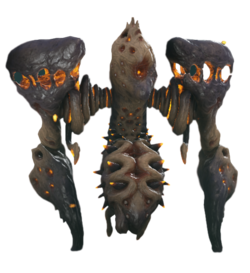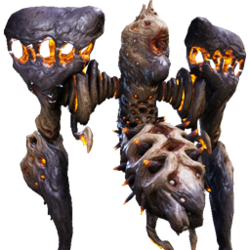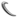+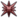++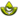+++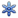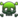−−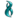−−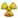−−−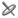++++−−

0 0

0 0 0 0 0 0 0 0 0

0 0 0 0 0 0 0 0 0 0 0 0

0 0 0 0 0 0 0

0 0 0 0

0

170
JavaScript未加载

MOD：

15 內融核心 1%

50 內融核心 0.28%

80 內融核心 0.04%

## 行动模式

• 与资料库描述不同，惊惧重岩者兼具远程与近身攻击方式。二者都可以造成很高的伤害。
• 远距离上，重岩者会打开肩膀上两个腔体中的一个，连续发射出数个带有跟踪效果的子弹，子弹没有撞击伤害，但命中任何表面后都会留下一片性的毒雾。
• 当玩家靠的太近时，重岩者会以两条肢体支撑地面，旋转身体进行致命的震地攻击。被击中的地面会出现一片性的区域。
• 在合适的距离上，重岩者会以两条肢体“起跳”，飞跃一大段距离，在飞行的过程中其躯干会不断旋转，同时向下发射多枚与第一种攻击中相同的子弹。这些子弹没有跟踪效果，会以轰炸的形式垂直击中地面，并在场景中留下大片的性毒雾。
• 重岩者自带机制复杂的减伤，但其肩膀上的两个腔体是其弱点。
• 两个腔体具有独立的生命值，且它们对伤害的减免程度明显不如躯干部分。
• 当破坏一个腔体后，躯干的减伤不会发生变化，但另一个腔体会受到更多的伤害。
• 攻击腔体被破坏后的部分可以直接对本体生命值造成伤害。
• 当破坏掉一个腔体时，对该被破坏的腔体处造成伤害，与腔体完全被破坏时相同，基本跟没有减伤造成的效果相同。破坏掉第二个腔体时两个被破坏的腔体处也都与破坏掉一个腔体时相同。
• 当两个腔体都被破坏，躯干的减伤效果就会大幅下降。
• 惊惧重岩者免疫任何触发效果，也不会被浮空或击倒，仅有部分控制类能力(例如魔导封锁)可以暂时压制住它。一旦两个腔体都被破坏，其对触发效果的抗性就消失了。除了以外的触发效果均可对其生效。
• 当一个腔体被破坏，其“伤口”部分会不断洒出腐蚀性的液体，就如同夜灵被击破的关节以及Lephantis被破坏的头部所留下的伤口一样。
• 脱离战斗10s后，重岩者会以每秒2%最大生命值的速度缓慢回复生命值。

## 伤害减免

\begin{aligned} DPS=(加成伤害)\cdot(加成射速)\cdot(加成多重射击)\cdot(部位倍率) \end{aligned}

$一般伤害减免= \begin{cases} 1,\; & DPS_{Average} \leq 1000 \\ 0.8 + \frac{200}{DPS_{Average}},\; & 1000 < DPS_{Average} \leq 2500 \\ 0.7 + \frac{450}{DPS_{Average}},\; & 2500 < DPS_{Average} \leq 5000 \\ 0.4 + \frac{1950}{DPS_{Average}},\; & 5000 < DPS_{Average} \leq 10000 \\ 0.2 + \frac{3950}{DPS_{Average}},\; & 10000 < DPS_{Average} \leq 20000 \\ 0.1 + \frac{5950}{DPS_{Average}},\; & 20000 < DPS_{Average} \end{cases}$

\begin{aligned} 非暴击DPS_{触发} & = (触发伤害)\cdot(1)\cdot(加成射速)\cdot(加成多重射击) \\ 暴击DPS_{触发} & = (触发伤害)\cdot(1+(暴击倍率-1)\cdot(暴击等级))\cdot(加成射速)\cdot(加成多重射击) \end{aligned}

$触发伤害减免= \begin{cases} 0.64,\; & DPS_{触发} \leq 1562.5 \\ 0.512 + \frac{200}{DPS_{触发}},\; & 1562.5 < DPS_{触发} \leq 3906.25 \\ 0.448 + \frac{450}{DPS_{触发}},\; & 3906.25 < DPS_{触发} \leq 7812.5 \\ 0.256 + \frac{1950}{DPS_{触发}},\; & 7812.5 < DPS_{触发} \leq 15625 \\ 0.128 + \frac{3950}{DPS_{触发}},\; & 15625 < DPS_{触发} \leq 31250 \\ 0.064 + \frac{5950}{DPS_{触发}},\; & 31250 < DPS_{触发} \end{cases}$

## 附注

• 惊惧重岩王者在某些时候会显示成惊惧重岩胸腔，而惊惧重岩者在某些时候会显示成重岩者股骨
• 重岩者的各种攻击都伴随着强烈的粒子效果。即使是在平静时，其肩膀上的两个腔体周围也有红色粒子漂浮。
• 重岩者唾液的卡面图片可以猜测，其肩膀上的两个腔体其实是其的口腔。
• 由于在破坏掉腔体之后，腔体处会一直不断洒出腐蚀性爆炸物，抛射范围较大，伤害较高，而且其拥有回复生命值的能力。推荐在击破腔体之后迅速将其消灭。

## 更新历史

• 修正了股骨被破坏前惊惧重岩者会被触发效果影响的问题。 股骨被破坏后重岩者才会被触发效果影响。

• 修正了非主机方玩家上惊惧重岩者会堆叠视觉特效的问题--现在应该能更容易看到这些家伙周围的情况了！

0.0
0人评价